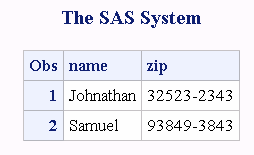# PRXMATCH Function

Searches for a pattern match and returns the position at which the pattern is found.

 Category: Character String Matching

## Syntax

PRXMATCH (, source)

### Required Arguments

#### regular-expression-id

specifies a numeric variable with a value that is a pattern identifier that is returned from the PRXPARSE function.

 Restriction If you use this argument, you must also use the PRXPARSE function.

#### perl-regular-expression

specifies a character constant, variable, or expression with a value that is a Perl regular expression.

#### source

specifies a character constant, variable, or expression that you want to search.

## Details

### The Basics

If you use regular-expression-id, then the PRXMATCH function searches source with the regular-expression-id that is returned by PRXPARSE, and returns the position at which the string begins. If there is no match, PRXMATCH returns a zero.
If you use perl-regular-expression, PRXMATCH searches source with the perl-regular-expression, and you do not need to call PRXPARSE.
You can use PRXMATCH with a Perl regular expression in a WHERE clause and in PROC SQL. For more information about pattern matching, see Pattern Matching Using Perl Regular Expressions (PRX) .

### Compiling a Perl Regular Expression

If perl-regular-expression is a constant or if it uses the /o option, then the Perl regular expression is compiled once and each use of PRXMATCH reuses the compiled expression. If perl-regular-expression is not a constant and if it does not use the /o option, then the Perl regular expression is recompiled for each call to PRXMATCH.
Note: The compile-once behavior occurs when you use PRXMATCH in a DATA step, in a WHERE clause, or in PROC SQL. For all other uses, the perl-regular-expression is recompiled for each call to PRXMATCH.

## Comparisons

The Perl regular expression (PRX) functions and CALL routines work together to manipulate strings that match patterns. To see a list and short description of these functions and CALL routines, see the Character String Matching category in .

## Examples

### Example 1: Finding the Position of a Substring by Using PRXPARSE

The following example searches a string for a substring, and returns its position in the string.
```/* For 9.0: the following example makes a call to PRXPARSE. */
/* For 9.1, no call is required. */ ```
```  data _null_;
/* Use PRXPARSE to compile the Perl regular expression.    */
patternID = prxparse('/world/');
/* Use PRXMATCH to find the position of the pattern match. */
position=prxmatch(patternID, 'Hello world!');
put position=;
run;```
SAS writes the following line to the log:
```   position=7
```

### Example 2: Finding the Position of a Substring by Using a Perl Regular Expression

The following example uses a Perl regular expression to search a string (Hello world) for a substring (world) and to return the position of the substring in the string.
```data _null_;
/* Use PRXMATCH to find the position of the pattern match. */
position=prxmatch('/world/', 'Hello world!');
put position=;
run;```
SAS writes the following line to the log:
```   position=7
```

### Example 3: Finding the Position of a Substring in a String: A Complex Example

The following example uses several Perl regular expression functions and a CALL routine to find the position of a substring in a string.
```data _null_;
if _N_ = 1 then
do;
retain PerlExpression;
pattern = "/(\d+):(\d\d)(?:\.(\d+))?/";
PerlExpression = prxparse(pattern);
end;

array match \$ 8;
input minsec \$80.;
position = prxmatch(PerlExpression, minsec);
if position ^= 0 then
do;
do i = 1 to prxparen(PerlExpression);
call prxposn(PerlExpression, i, start, length);
if start ^= 0 then
match[i] = substr(minsec, start, length);
end;
put match "minutes, " match "seconds" @;
if ^missing(match) then
put ", " match "milliseconds";
end;
datalines;
14:56.456
45:32
;
run;```
The following lines are written to the SAS log:
```   14 minutes, 56 seconds, 456 milliseconds
45 minutes, 32 seconds```

### Example 4: Extracting a ZIP Code by Using the DATA Step

The following example uses a DATA step to search each observation in a data set for a nine-digit ZIP code, and writes those observations to the data set ZipPlus4.
```data ZipCodes;
input name: \$16. zip:\$10.;
datalines;
Johnathan 32523-2343
Seth 85030
Kim 39204
Samuel 93849-3843
;
/* Extract ZIP+4 ZIP codes with the DATA step. */
data ZipPlus4;
set ZipCodes;
where prxmatch('/\d{5}-\d{4}/', zip);
run;
proc print data=ZipPlus4;
run;```
ZIP Code Output from the DATA Step### Example 5: Extracting a ZIP Code by Using PROC SQL

The following example searches each observation in a data set for a nine-digit ZIP code, and writes those observations to the data set ZipPlus4.
```data ZipCodes;
input name: \$16. zip:\$10.;
datalines;
Johnathan 32523-2343
Seth 85030
Kim 39204
Samuel 93849-3843
;
/* Extract ZIP+4 ZIP codes with PROC SQL. */
proc sql;
create table ZipPlus4 as
select * from ZipCodes
where prxmatch('/\d{5}-\d{4}/', zip);
run;
proc print data=ZipPlus4;
run;```
ZIP Code Output from PROC SQL